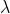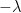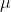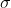In: Math

# A recent Pew Center Research survey revealed that 68% of high school students have used tobacco...

A recent Pew Center Research survey revealed that 68% of high school students have used tobacco related products. Suppose a statistician randomly selected 20 high school students. Use this information to answer questions 39-41.

1. To find the probability that exactly 15 used tobacco products, would you use the Binomial, Geometric, or Poisson distribution to find the probability?
2. Set up the problem to find P (X = 15) using the appropriate distribution. Do NOT solve.
3. What is the expected number of high school students in the sample who have used tobacco products?

For a self check out at the local Walmart, the mean number of customers per 5 minute interval is 1.5 customers. Use this information to answer questions 42 and 43.

1. To find the probability of 2 customers in the next 5 minutes, would you use the Binomial, Geometric, or Poisson distribution to find the probability?
2. What is the variance (numeric value)?

Assuming the grades on the first homework are nearly normal with N(90, 4.3), what proportion of grades fall between 85 and 90?
Assuming the grades on the final exam are nearly normal with N(90, 4.3), for a grade of 95 or more on the exam, find the z-score and explain what it means.
Assuming the grades on the final exam are nearly normal with N(90, 4.3), what is the minimum grade putting you in the top 15% of the class?
Assuming the grades on the final exam are nearly normal with N(82, 3.86), what proportion of grades fall between 85 and 90?

## Solutions

##### Expert Solution

1) This is a binomial probability distribution

p = 0.68

n = 20

P(X = x) = nCx * px * (1 - p)n - x

P(X = 15) = 20C15 * (0.68)^15 * (0.32)^5 = 0.1599

Expected number of students = np = 20 * 0.68 = 13.6

2) This is a poisson distribution.= 1.5

P(X = x) = e/x!

P(X = 2) = e^(-1.5) * (1.5)^2/2! = 0.2510

Variance == 1.5

3) P(85 < X < 90)

= P((85 -)/< (X -)/< (90 -)/)

= P((85 - 90)/4.3 < Z < (90 - 90)/4.3)

= P(-1.16 < Z < 0)

= P(Z < 0) - P(Z < -1.16)

= 0.5 - 0.1230

= 0.3770

4) z-score = (X -)/= (95 - 90)/4.3

= 1.16

The grade score 95 is 1.16 standard deviation above the mean.

5) P(X > x) = 0.15

or, P((X -)/> (x -)/) = 0.15

or, P(Z > (x - 90)/4.3) = 0.15

or, P(Z < (x - 90)/4.3) = 0.85

or, (x - 90)/4.3 = 1.04

or, x = 1.04 * 4.3 + 90

or, x = 94.472

6) P(85 < X < 90)

= P((85 -)/< (X -)/< (90 -)/)

= P((85 - 82)/3.86 < Z < (90 - 82)/3.86)

= P(0.78 < Z < 2.07)

= P(Z < 2.07) - P(Z < 0.78)

= 0.9808 - 0.7823

= 0.1985

## Related Solutions

##### In a recent survey conducted by the Pew Research Center, a random sample of adults 18...
In a recent survey conducted by the Pew Research Center, a random sample of adults 18 years of age or older living in the continental united states was asked their reaction to the word socialism. In addition, the individuals were asked to disclose which political party they most associate with. Results of the survey are given in the table. Democrat Independent Republican Positive 220 144 62 Negative 279 410 351 a) Explain why this data should be analyzed by the...
##### 1: A survey questioned 1,000 high school students. The survey revealed that 46% are honor roll...
1: A survey questioned 1,000 high school students. The survey revealed that 46% are honor roll students. Of those who are honor roll students, 45% play sports in school and 21% of those that are not honor roll students, don't play sports. What is the probability that a high school student selected at random plays sports in school? 2: One of two small classrooms is chosen at random with equally likely probability, and then a student is chosen at random...
##### In a recent survey of high school students, it was found that the amount of time...
In a recent survey of high school students, it was found that the amount of time spent on reading books per week was normally distributed with a mean of 32 minutes. Assume the distribution of weekly reading time follows the normal distribution with a population standard deviation of 2 minutes. Suppose we select a sample of 20 high school students. a. What is the standard error of the mean time? Answer b. What percent of the sample means will be...
##### According to a recent Pew Research Center survey, 231 out of 371 Americans surveyed with just...
According to a recent Pew Research Center survey, 231 out of 371 Americans surveyed with just a high school diploma and 543 out of 634 Americans surveyed with a college degree have read at least one book in the last 12 months. Based on these sample results and a 0.01 level of significance, can one infer that the proportion of Americans who have read at least one book in the last 12 months is higher for those with a college...
##### A survey of high school students revealed that the numbers of soft drinks consumed per month...
A survey of high school students revealed that the numbers of soft drinks consumed per month was normally distributed with mean 25 and standard deviation 15. A sample of 36 students was selected. What is the probability that the average number of soft drinks consumed per month for the sample was between 26.2 and 30 soft drinks? Write only a number as your answer. Round to 4 decimal places (for example 0.0048). Do not write as a percentage.
##### A survey of high school students revealed that the numbers of soft drinks consumed per month...
A survey of high school students revealed that the numbers of soft drinks consumed per month was normally distributed with mean 25 and standard deviation 15. A sample of 36 students was selected. What is the probability that the average number of soft drinks consumed per month for the sample was between 28.5 and 30 soft drinks? Write only a number as your answer. Round to 4 decimal places (for example 0.0048). Do not write as a percentage.
##### A survey of high school students revealed that the numbers of soft drinks consumed per month...
A survey of high school students revealed that the numbers of soft drinks consumed per month was normally distributed with mean 25 and standard deviation 15. A sample of 36 students was selected. What is the probability that the average number of soft drinks consumed per month for the sample was between 28.1 and 30 soft drinks? Write only a number as your answer. Round to 4 decimal places (for example 0.0048). Do not write as a percentage.
##### The Pew Research Center Internet Project conducted a survey of 657 Internet users. This survey provided...
The Pew Research Center Internet Project conducted a survey of 657 Internet users. This survey provided a variety of statistics on them. If required, round your answers to four decimal places. (a) The sample survey showed that 90% of respondents said the Internet has been a good thing for them personally. Develop a 95% confidence interval for the proportion of respondents who say the Internet has been a good thing for them personally. ______ to _____ (b) The sample survey...
##### The Pew Research Center Internet Project conducted a survey of 757 Internet users. This survey provided...
The Pew Research Center Internet Project conducted a survey of 757 Internet users. This survey provided a variety of statistics on them. If required, round your answers to four decimal places. (a) The sample survey showed that 90% of respondents said the Internet has been a good thing for them personally. Develop a 95% confidence interval for the proportion of respondents who say the Internet has been a good thing for them personally. to (b) The sample survey showed that...
##### The Pew Research Center Internet Project conducted a survey of 857 Internet users. This survey provided...
The Pew Research Center Internet Project conducted a survey of 857 Internet users. This survey provided a variety of statistics on them. If required, round your answers to four decimal places. (a) The sample survey showed that 90% of respondents said the Internet has been a good thing for them personally. Develop a 95% confidence interval for the proportion of respondents who say the Internet has been a good thing for them personally. 0.880 to 0.920 (b) The sample survey...